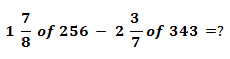# SBI Clerk Prelims 2021 Quantitative Aptitude Questions (Day-06)

Dear Aspirants, Our IBPS Guide team is providing new series of Quantitative Aptitude Questions for SBI Clerk Prelims 2020 so the aspirants can practice it on a daily basis. These questions are framed by our skilled experts after understanding your needs thoroughly. Aspirants can practice these new series questions daily to familiarize with the exact exam pattern and make your preparation effective.

Start Quiz

Simplification

Directions (1-5): What value should come in the place of (?) in the following questions?

1)A.– 453

B.– 353

C.– 383

D.– 473

E.None of these

2) (8)2.8 * (2)1.6 *(?) = (16)3/2/(64)1/2

A. 29

B. 1/27

C.1/2-7

D.1/29

E.None of these

3) (2197)1/3 + 44 – (512)1/3 = (?)2

A.9

B.7

C.8

D.13

E.None of these

4) 45% of 1800 – 456 + 24% of 1500 + 324 =?

A.1156

B.1224

C.1024

D.1038

E.None of these

5)
95 * 85 – 5698 ÷ 7 = (?)*5

A.1562.65

B.1489.20

C.1452.20

D.1123.60

E.None of these

Caselet

Directions (6-10):  Study the following information carefully and answer the questions given below.

Three persons Ravi, Ram and Sundar spend 8%, 10% and 12% of their monthly income on food and house rent. Each of them saves half of the remaining amount. The monthly income of Ravi and Sundar is same and the monthly savings of Ravi is Rs.500 more than that of Sundar. The total expenditure of Ravi and Ram together on food and house rent is Rs.1400 more than that of Sundar.

6) What is the monthly expenditure of Ravi and Ram together on food and house rent?

A.Rs.4000

B.Rs.4200

C.Rs.4400

D.Rs.4600

E.None of these

7) The monthly income of Ram is how much less than the monthly income of Sundar?

A.Rs.1000

B.Rs.500

C.Rs.1500

D.Rs.2000

E.None of these

8) What is the total amount saved by Ravi, Ram and Sundar in a month?

A.Rs.30000

B.Rs.31000

C.Rs.32000

D.Rs.33300

E.None of these

9) The total monthly savings of Ravi, Ram and Sundar is what percent of their total monthly income?

A.30%

B.45%

C.15%

D.40%

E.None of these

10) By how much should Ravi’s monthly income be increased so that the monthly expenditures of Ravi on food and house rent will become equal to Sundar?

A.Rs.12500

B.Rs.13000

C.Rs.14700

D.Rs.15260

E.None of these

= 15/8 * 256 – 17/7 *343

= 15*32 – 17*49

= 480 – 833 = -353

(23)2.8 * (2)1.6 * (?) = (24)3/2/(26)1/2

=> (2)8.4 * (2)1.6 * (?) = (2)4*3/2/(2)6*1/2

=> (2)8.4+1.6 *(?) = (2)6/(2)3

? = 23/210 = 2-7

(2197)1/3 + 44 – (512)1/3 = (?)2

(?)2= 13 + 44 – 8

49 = (?)2

?= 7

45% of 1800 – 456 + 24% of 1500 + 324 =?

=> 45/100 x 1800 – 456 + 24/100 x 1500 + 324 =?

=> 810 – 456 + 360 + 324 =?

=> 1038 =?

95 * 85 – 5698 ÷ 7 = (?)*5

8075 – 814 = (?) *5

7261/5 =?

1452.2 =?

Directions (6-10) :

Let the monthly income of Ravi = Rs.100x.

Monthly expenditure of Ravi = 8% of 100x = Rs.8x

Remaining = 100x – 8x = 92x

Savings by Ravi = 92x/2 = Rs.46x

Let the monthly income of Ram = Rs.100y.

Monthly expenditure = 10% of 100y = Rs.10y.

Remaining = 100y – 10y = 90y

Savings by Ram = 90y/2 = Rs.45y

Monthly income of Sundar = Monthly income of Ravi = Rs.100x

Monthly expenditure of Sundar = 12% of 100x = Rs.12x

Remaining = 100x – 12x = 88x

Savings by Sundar = 88x/2 = Rs.44x

Monthly savings of Ravi is Rs.500 more than that of Sundar.

46x – 44x = 2x = 500 => x = 250

Total expenditure of Ravi and Ram together is Rs.1400 more than that of Sundar.

8x + 10y = 1400 + 12x => y = 240

Total monthly expenditure of Ravi and Ram = 8x + 10y

= 8 * 250 + 10 * 240

= Rs.4400

Monthly income of Ram = 100y = 100 * 240 = 24000

Monthly income of Sundar = 100x = 100 * 250 = 25000

Required income = 25000 – 24000 = Rs.1000

Total savings = 46x + 45y + 44x

= 90x + 45y

= 90 * 250 + 45 * 240

= Rs.33300

Total income = 100x + 100y + 100x

= 200x + 100y

= 200 * 250 + 100 * 240

= Rs.74000

Total savings = 46x + 45y + 44x

= 90x + 45y

= Rs.33300

Required percentage = 33300/74000 * 100 = 45%

Monthly expenditure of Ravi = 8x = 2000

Monthly expenditure of Sundar = 12x = 3000

Let the monthly income of Ravi increased by a%.

8% of (100 + a)% of 100x = 3000

By solving this equation, we get a = 50%

Required amount = a% of 25000 = Rs.12500

 Check Here to View SBI Clerk Prelims 2021 Quantitative Aptitude Questions Day – 05 Day – 04 Day – 03